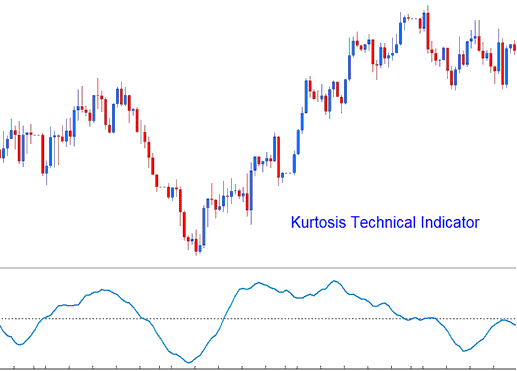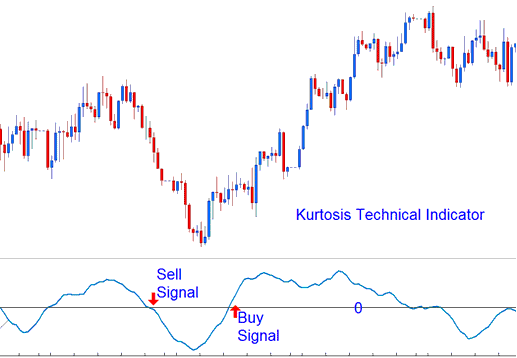# Kurtosis Forex Technical Analysis and Kurtosis Forex Trading Signals

This indicator is used to help determine the sentiment of the Forex market.

It is calculated in 3 parts.

• Kurtosis portion
• Fast Kurtosis
• Fast/Slow Kurtosis

The Kurtosis portion is calculated using the formula:

K = n-periods momentum – n-periods momentum of 1 bar before (current price bar’s momentum value minus the previous price bar’s momentum value)

The Fast Kurtosis portion is calculated using the formula:

FK = n-periods moving average of K

The Fast/Slow Kurtosis portion is calculated using the formula:

FSK = n-periods moving average of FK## Forex Technical Analysis and Generating Forex Trading Signals

Bullish Signal- A buy signal is generated when the indicator crosses above zero.

Bearish Signal- A sell signal is generated when the indicator crosses below zero.Technical Analysis

Limited Time 100% Account Opening Bonus - Open Account

Top 100 Forex Broker Rankings